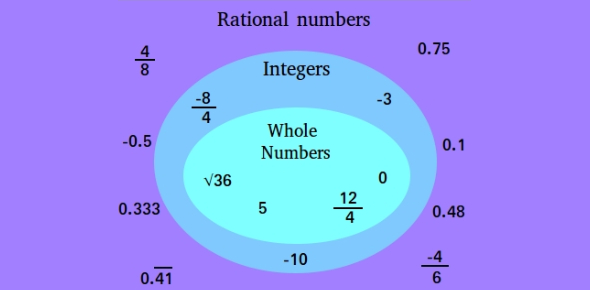# A Quiz On Rational Numbers! Trivia

8 Questions | Attempts: 8387SettingsAre you familiar with rational numbers? Do you suppose you can vanquish this quiz? A rational number is a figure that is equal to the quotient of two integers p and q and can be articulated as some fraction where the numerator and denominator are integers. Some examples of rational numbers include the number 8, as the fraction 8/1 and the fraction 5/7. Taking this quiz will assist you to see how much you know about rational numbers.

• 1.
2 1/4 converted to an improper fraction is:
• A.

7/4

• B.

6/4

• C.

4/9

• D.

9/4

• 2.
1/9 written as a decimal is _________.
• A.

0.1

• B.

0.01

• C.

_ 0.1

• D.

0.001

• 3.
The answer to Question 4 is known as a _________________ decimal.
• A.

Temporary

• B.

Registered

• C.

Terminating

• D.

Repeating

• 4.
Which list of rational numbers is correctly ordered from least to greatest?9 3/4, 9.74, 9 5/7, 9.72, 9 9/13
• A.

9 9/13, 9 5/7, 9.72, 9.74, 9 3/4

• B.

9 3/4, 9.72, 9 5/7, 9.74, 9 9/13

• C.

9.72, 9.74, 9 5/7, 9 3/4, 9 9/13

• D.

9.72, 9.74, 9 3/4, 9 5/7, 9 9/13

• 5.
When a repeating decimal is converted to a fraction, the denominator will always be _______.
• A.

0

• B.

1

• C.

10

• D.

9

• 6.
Order the following rational numbers from least to greatest: -0.8, -3/4, 1.25, 0
• A.

0, -0.8, -3/4, 1.25

• B.

0, 1.25, -0.8, -3/4

• C.

-0.8, -3/4, 0, 1.25

• D.

1.25, 0, -3/4, -0.8

• 7.
Compare the following rational numbers and tell whether they are greater than, less than, or equal to each other. 4                   0.0410
• A.

• B.

>

• C.

=

• 8.
When a fraction is converted to a decimal through long division, a terminating decimal is formed when the remainder is __________________.
• A.

Less

• B.

Repeated

• C.

Zero

• D.Back to top